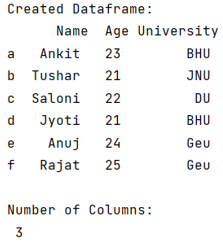# How to retrieve the number of columns in a Pandas DataFrame?

Given a Pandas DataFrame, we have to retrieve the number of columns. By Pranit Sharma Last updated : September 22, 2023

Pandas is a special tool that allows us to perform complex manipulations of data effectively and efficiently. Inside pandas, we mostly deal with a dataset in the form of DataFrame. DataFrames are 2-dimensional data structures in pandas. DataFrames consist of rows, columns, and the data.

## Retrieving the number of columns in a Pandas DataFrame

There are several ways to retrieve the number of columns in a pandas DataFrame. The len(df.columns) method is a better way to retrieve the number of columns.

Note

To work with pandas, we need to import pandas package first, below is the syntax:

```import pandas as pd
```

Let us understand with the help of an example,

## Python program to retrieve the number of columns in a Pandas DataFrame

```# Import pandas Package
import pandas as pd

# Creating dictionary
d = {
'Name':['Ankit', 'Tushar', 'Saloni','Jyoti', 'Anuj', 'Rajat'],
'Age':[23, 21, 22, 21, 24, 25],
'University':['BHU', 'JNU', 'DU', 'BHU', 'Geu', 'Geu']
}

# Creating a Dataframe
df = pd.DataFrame(d,index = ['a', 'b', 'c', 'd', 'e', 'f'])

print("Created Dataframe:\n", df,"\n")

# Display total number of columns
Columns = len(df.columns)

print("Number of Columns:\n",Columns)
```

### Output

The output of the above program is: